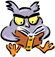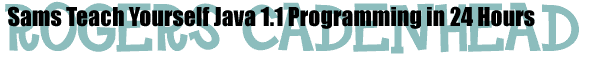Hours with Activities on this Page: 4 | 5 | 6 | 7 | 9 | 11 | 18 | 20 | 22.

## Activities

Each Hour of the book includes suggested activities related to the subject matter. These are offered as ideas to encourage readers to expand their knowledge and build up some practice with Java.

As time permits, source code will be added here to show how some of the activities can be accomplished.

### Hour 4

• Page 58: The second activity suggested in this hour is implemented in a Java applet called TwoParameters.java. You can also see the applet running on a Web page.

### Hour 5

• Page 75: This hour's first activity is implemented in a Java application called ElvisPlus.java.

### Hour 6

• Page 88: The second activity suggested in this hour is implemented in a Java applet called UpperCredits.java.

### Hour 7

• Page 102: The second activity suggested in this hour is implemented in two applications: NoteGrade1.java and NoteGrade2.java. The first uses a series of `if` statements while the second uses a `switch` statement. Because `switch` only can handle a single value in each `case` statement, this activity is more efficient using a series of `if` statements.

### Hour 9

• Page 126: The first activity suggested in this hour is implemented in a Java class called GradeStudents.java. A multidimensional array of integers is created to store the grades of six students, and the average grade of each student, and the average grade of all students, is determined.

• Page 126: The second activity suggested in this hour is implemented in a Java class called Find400Primes.java. To find out if a number is prime, you have to use the `%` operator in Java, which returns the remainder of a division operation. If you divide a number x by every number from 2 through the square root of x, any division that has a 0 remainder means that x is not prime. This can be used to find the first 400 prime numbers. for more information on primes, visit the Prime Page.

### Hour 11

• Page 151: The activity suggested in this hour is implemented in a Java class called NewVirus.java, and you can test it with a Java class called TestNewVirus.java.

### Hour 18

• Page 240: The first activity suggested in this hour is implemented in a Java class called NewBounce.java, which is an expansion of the Bounce.java program featured during the hour. You can also see the applet running on a Web page. Note: Although the book recommends that the `height` variable should be modified for this activity, a better solution requires the creation of a new variable called `strength`. `strength` equals 1 when the bounce is at full strength, and decreases by 10 percent every time the ball hits the ground. The following statement makes this decrease occur:

• ```strength = (float)(strength * 0.9);```

The `strength` variable can then be used as a multiplier each time the bounce's height is calculated:

• ```double bounce = Math.sin(current) * height * strength;```

### Hour 20

• Page 269: The second activity suggested in this hour is implemented in a Java class called TextMadness.java. The TextListener interface is added to the class, an addTextListener method is used for each element of the `numbers` array, and a `textValueChanged` method is called automatically whenever the user changes the numbers used for drawings. If your Web browser supports Java 1.1, you can see the applet running on a Web page.

### Hour 22

• Page 299: The second activity suggested in this hour is implemented in MultiCraps.java (you also need Die.class from the Craps project and a simple Web page like multicraps.html). In order to roll the dice multiple times, a helper class is created that can be used as a thread. This class has the job of rolling the dice over and over again until it (the thread) is killed.